# RD Sharma Solutions Class 12 Differential Equations Exercise 22.1

RD Sharma Solutions for Class 12 Maths Exercise 22.1 Chapter 22 Differential Equations covers all the exercise questions in the RD Sharma textbook. You can download this as a free pdf. These RD Sharma Solutions for Class 12 Maths Chapter 22 are prepared by our well-trained and qualified subject experts and are provided here to assist students in learning the concepts quickly.

## Download PDF of RD Sharma Solutions for Class 12 Maths Chapter 22 Exercise 1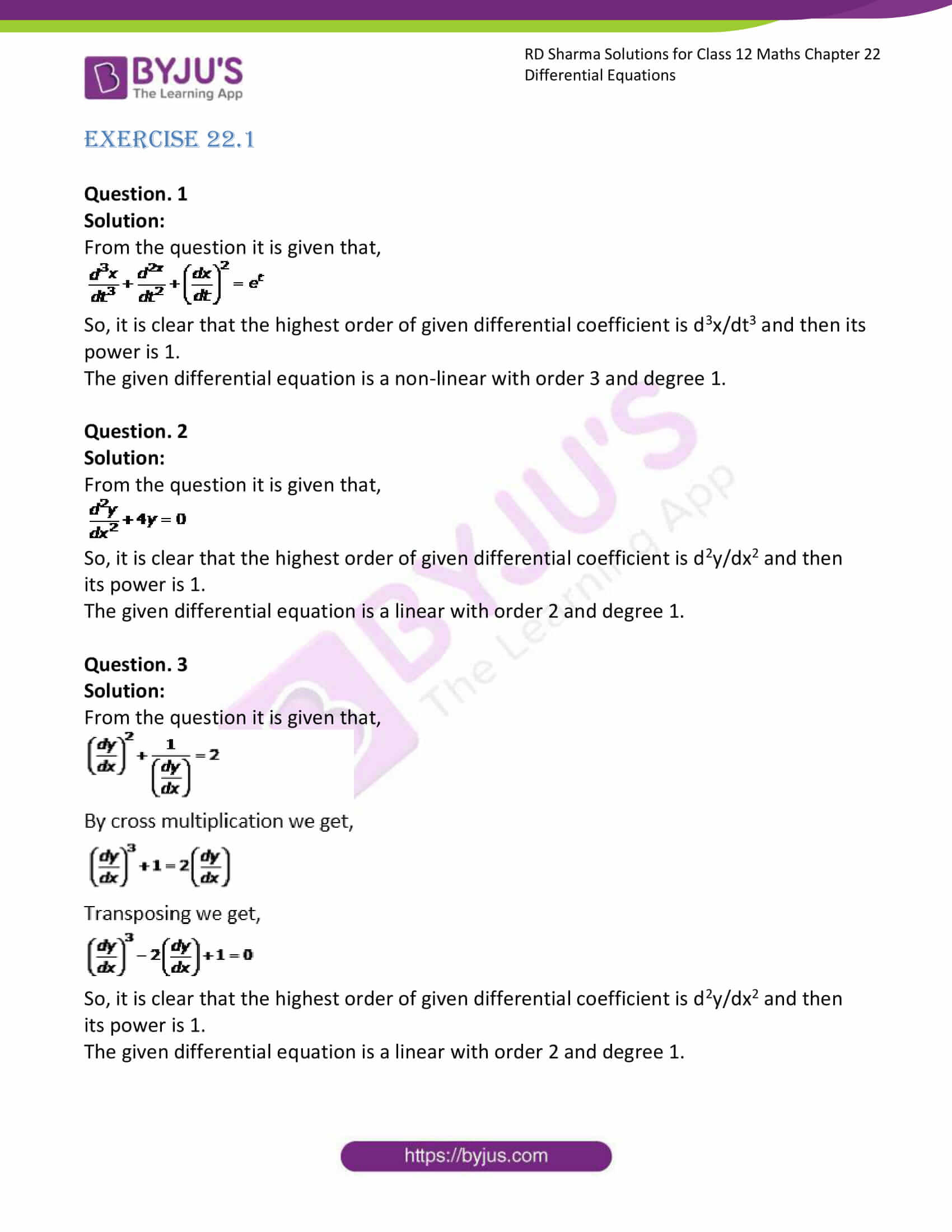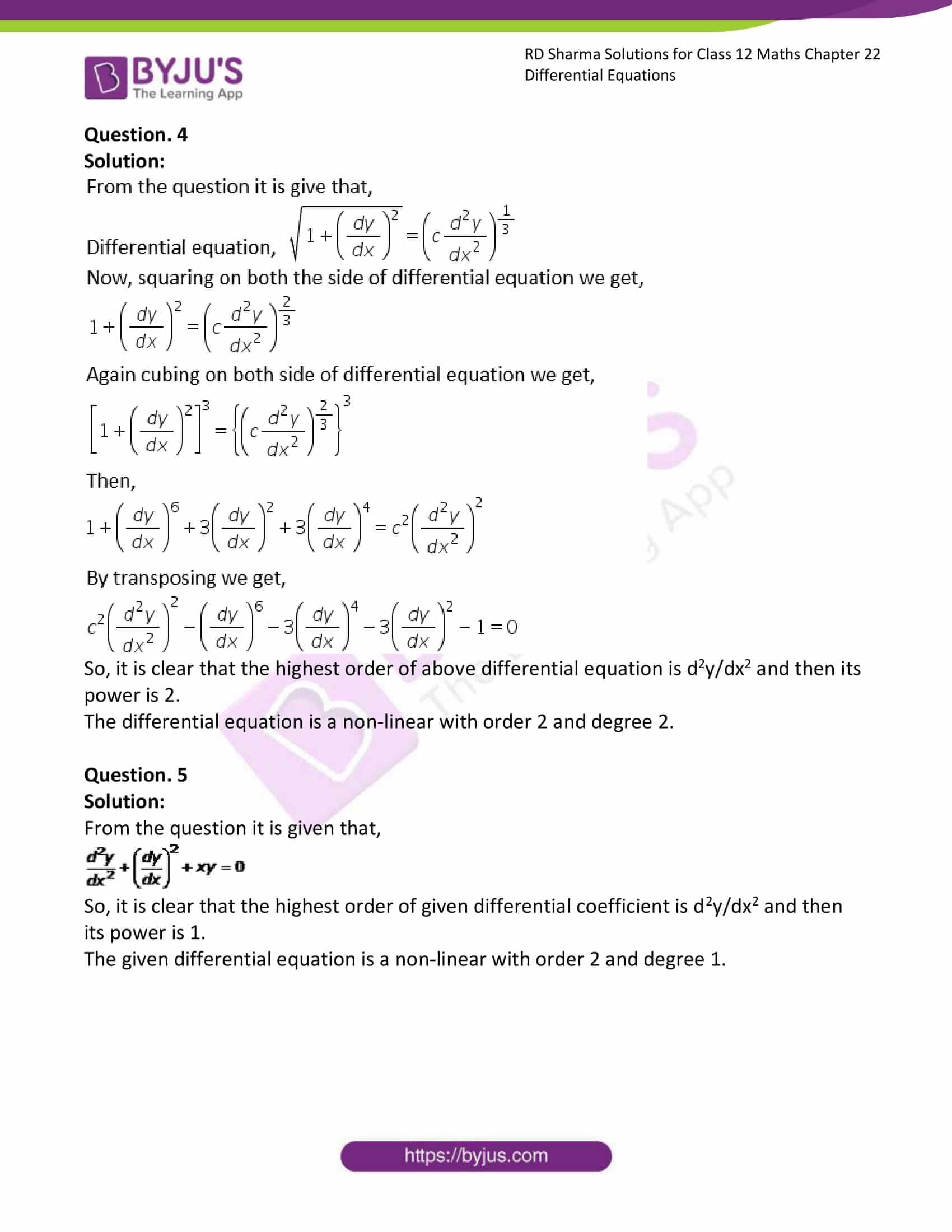### Access RD Sharma Solutions for Class 12 Maths Chapter 22 Exercise 1

EXERCISE 22.1

Question. 1

Solution:

From the question it is given that,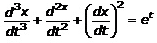So, it is clear that the highest order of given differential coefficient is d3x/dt3 and then its power is 1.

The given differential equation is a non-linear with order 3 and degree 1.

Question. 2

Solution:

From the question it is given that,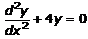So, it is clear that the highest order of given differential coefficient is d2y/dx2 and then its power is 1.

The given differential equation is a linear with order 2 and degree 1.

Question. 3

Solution:

From the question it is given that,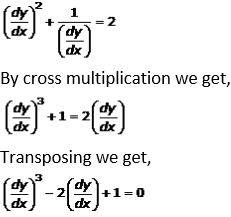So, it is clear that the highest order of given differential coefficient is d2y/dx2 and then its power is 1.

The given differential equation is a linear with order 2 and degree 1.

Question. 4

Solution: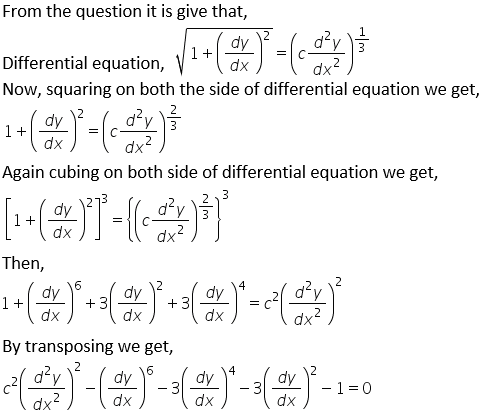So, it is clear that the highest order of above differential equation is d2y/dx2 and then its power is 2.

The differential equation is a non-linear with order 2 and degree 2.

Question. 5

Solution:

From the question it is given that,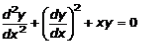So, it is clear that the highest order of given differential coefficient is d2y/dx2 and then its power is 1.

The given differential equation is a non-linear with order 2 and degree 1.